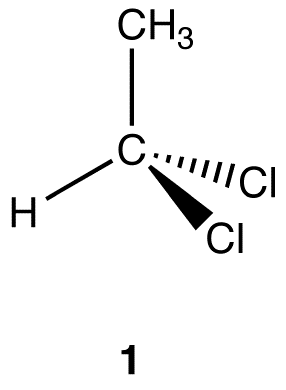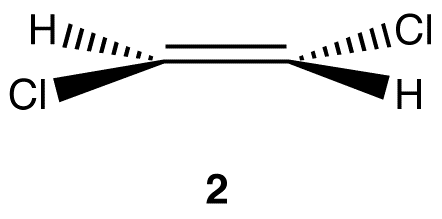Plane of Symmetry

•• Contributed by Gamini Gunawardena
• Associate Professor (Chemistry) at Utah Valley University

A plane of symmetry is an imaginary plane that bisects a molecule into halves that are mirror images of each other.

eg. 1:In 1, the vertical plane that bisects the methyl group, the carbon atom, and the hydrogen atom bisects the molecule into halves that are mirror images of each other. Therefore, it is a plane of symmetry.

eg. 2:In 2, the horizontal plane that bisects all six atoms in the molecule bisects the molecule into halves that are mirror images of each other. Therefore, it is a plane of symmetry.

eg. 3:In 3, the vertical plane that passes through the red broken line perpendicular to the plane of the cyclopropane ring bisects the molecule into halves that are mirror images of each other. Therefore, it is a plane of symmetry.